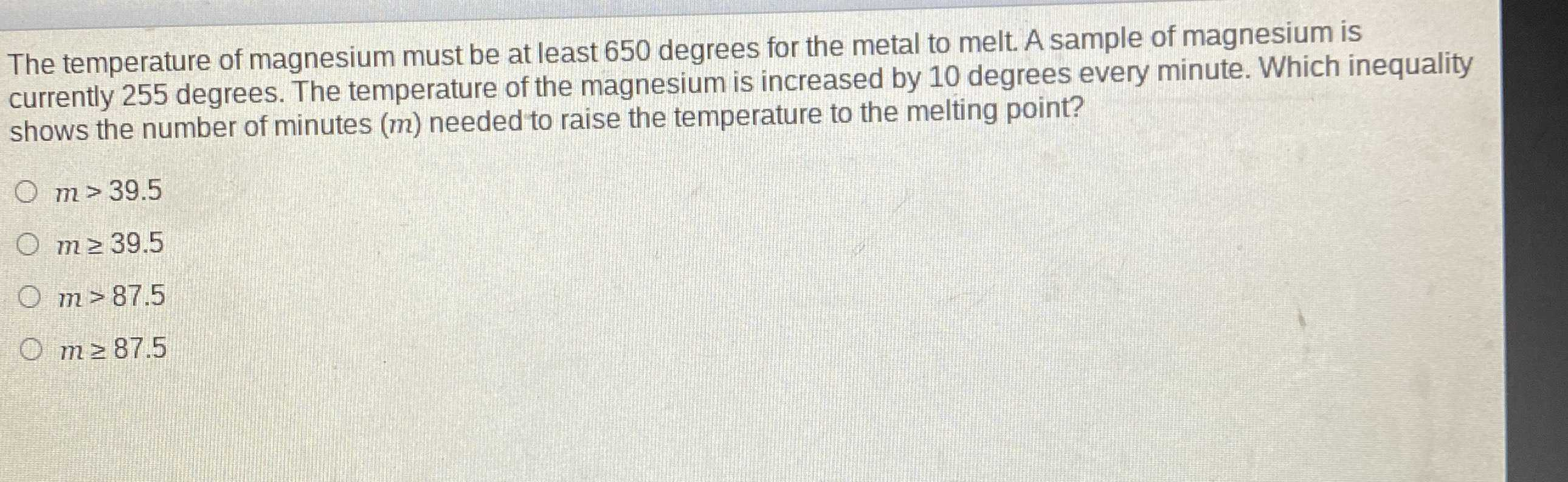### Still have math questions?

Algebra
QuestionThe temperature of magnesium must be at least $$650$$ degrees for the metal to melt. A sample of magnesium is currently $$255$$ degrees. The temperature of the magnesium is increased by $$10$$ degrees every minute. Which inequality shows the number of minutes $$( m )$$ needed to raise the temperature to the melting point?

$$m > 39.5$$

$$m \geq 39.5$$

$$m > 87.5$$

$$m \geq 87.5$$